#SPE1 Example

## Set up gravity and add IMPES solver and deckformat.

```gravity reset
gravity on

```

## Initialize the model bases on "ODEH.data"

We read in the deck file, convert the units to SI and then use the resulting deck variable to create grid, rock, fluid and well configurations.

```current_dir = fileparts(mfilename('fullpath'));
fn    = fullfile(current_dir, 'data', 'ODEH.DATA');

deck  = convertDeckUnits(deck);
fluid = initEclipseFluid(deck);
rock  = initEclipseRock(deck);
G     = initEclipseGrid(deck);
G     = computeGeometry(G);

% Remove any rock data corresponding to inactive cells.
rock  = compressRock(rock, G.cells.indexMap);
state = initEclipseState(G, deck, fluid);

% Since we are going to solve the pressure using a TPFA scheme, we should
% use the 'ip_tpf' inner product when reading in wells.
wells = processWells(G, rock, deck.SCHEDULE.control, ...
'InnerProduct', 'ip_tpf');

% Set up well solutions
initP = state.pressure(1);
state.wellSol = initWellSol(wells, initP);

% Plot grid and wells
clf;
plotGrid(G, 'FaceAlpha', .3, 'EdgeAlpha', .1);
plotWell(G, wells);
view(-20, 30);
axis tight;
```
```@ The black-oil model currently implemented does not support slower than
@ instantaneous dissolution of gas into oil.

Warning: Keyword DRSDT is currently ignored in MRST blackoil codes.
Warning: The maximum gas saturation (=1.00) should equal 1-(connate water saturation) (=0.88).
```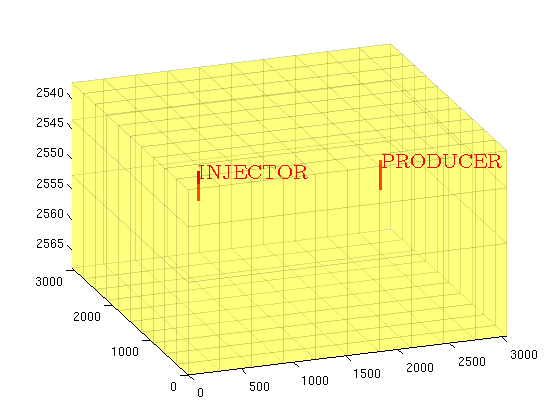## Compute various quantities needed for the IMPES solver

```% Wells are the only driving forces, giving no-flow along the boundary.
forces = {'bc', [], 'src', [], 'wells', wells};

porvol = poreVolume(G, rock);
TSTEP  = convertFrom(spe1_dt, day);
htrans = computeTrans(G, rock);
state  = computeFacePressure(state, G, htrans, fluid);
Trans  = computeTrans(G, rock);
```

## Solve the model

```start  = deck.RUNSPEC.START;
nstep = numel(TSTEP); T = 0;
report = [];

fprintf('Simulating %1.0f days of production in %d time steps...\n',...
convertTo(sum(TSTEP), day), numel(TSTEP))

for k = 1 : nstep,
DT = TSTEP(k);

% Solve pressure and transport
[state, dt, report] = ...
impesTPFA(state, G, Trans, fluid, DT, porvol, forces{:},       ...
'ATol', 5.0e-5, 'RTol', 5.0e-13,                     ...
'EstimateTimeStep', false, 'DynamicMobility', false, ...
'report', report);

% Plot gas saturation.
clf;
subplot(2,1,1)
plotCellData(G, state.s(:,3));
view(-20, 30);
colorbar
caxis([0 0.5])
axis tight off
title(sprintf('Gas saturation after %1.0f days', convertTo(T, day())))

% Plot pressure
subplot(2,1,2)
plotCellData(G, state.pressure);
view(-20, 30);
colorbar
axis tight off
title(sprintf('Pressure after %1.0f days', convertTo(T, day())))

drawnow

% Update for next time step
T = T + DT;
end
```
```Simulating 1216 days of production in 151 time steps...

```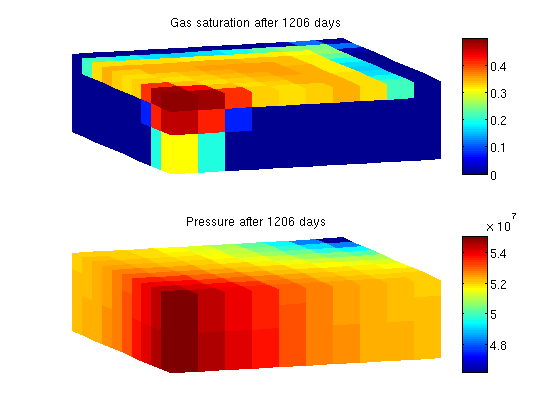## Plot bottom hole pressure for the wells

```clf;
plot(convertTo([report.TIME], day), [report.WBHP], '-.')
legend({wells.name}, 'Location', 'Best')
xlabel('Days')
ylabel('bar')
```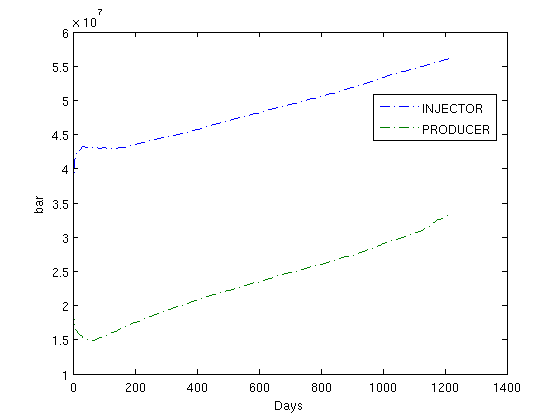## Plot producer Gas/Oil ratio

```clf;
plot(convertTo([report.TIME], day), [report.WGOR])
title('WGOR')
xlabel('Days')
```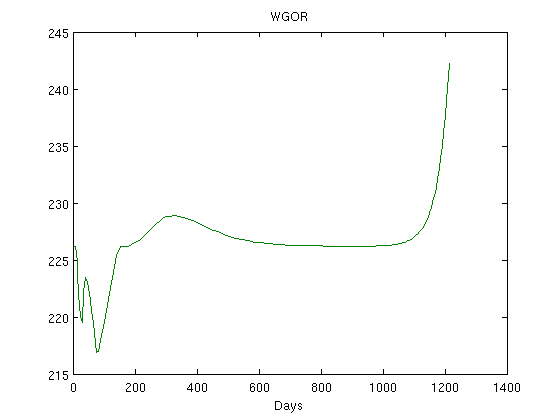## Plot accumulated production

```t = [0, report.TIME];

clf
subplot(1,2,1)
% Plot accumulated gas production total at the producer
wgpr = [report.WGPR];
wgpr = [0, wgpr(2,:)];
wgpt = cumtrapz(t, wgpr);
plot(convertTo(t,day), convertTo(wgpt, 1e6*meter^3))
title('WGPT')
ylabel('MM m^3')
xlabel('Days')

subplot(1,2,2)
% Plot accumulated oil production total at the producer
wopr = [report.WOPR];
wopr = [0, wopr(2,:)];
wopt = cumtrapz(t, wopr);
plot(convertTo(t, day), convertTo(wopt, 1e6*stb))
title('WOPT')
ylabel('MM stb')
xlabel('Days')
```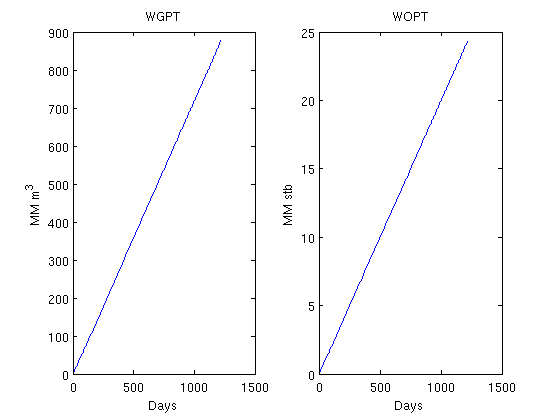Published October 2, 2012